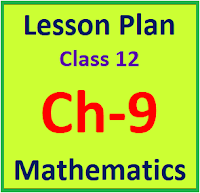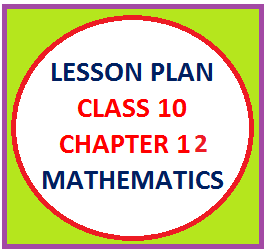## Posts

Showing posts from February, 2020

### Resource Centre Mathematics

Resource Centre Mathematics Mathematics worksheet, mathematics basic points and formulas, mathematics lesson plan, mathematics multiple choice questions Workplace Dashboard CBSE Syllabus For Session 2023-24 For  :   Classes IX & X    |   Classes XI & XII Watch Videos on Maths Solutions CLASS IX MATHEMATICS FORMULAS &  BASIC CONCEPTS

### Lesson Plan Math Class XII Ch-12 | Linear ProgrammingE- LESSON PLAN    SUBJECT MATHEMATICS     CLASS 10+2 Lesson Plan, Class XII Subject Mathematics, chapter XII, Linear Programming Problems , for Mathematics Teacher. Effective way of Teaching Mathematics. Top planning by the teacher for effective teaching in the class. E lesson planning for mathematics. Board – CBSE CLASS –XII SUBJECT- MATHEMATICS CHAPTER 12  :- Linear Programming TOPIC:- CHAPTER 12 : Linear Programming DURATION:-    This chapter is divided into 10 modules and is completed in 15 class meetings. PRE- REQUISITE KNOWLEDGE:- Knowledge of Cartesian coordinate system to represent the graph Knowledge of representing linear inequalities on the graph and method to find their solution (Chapter 6 class XI) TEACHING AIDS:-   Green Board, Chalk,   Duster, Charts, smart board, projector, laptop etc. METHODOLOGY:-    Lecture method OBJECTIVES:- Introduction of Linear Programming Relat

### Lesson Plan Math Class XII Ch- 9 | Differential EquationsE- LESSON PLAN     SUBJECT MATHEMATICS      CLASS 10+2 Lesson Plan, Class XII Subject Mathematics, chapter 9, Differential Equations , for Mathematics Teacher. Effective way of Teaching Mathematics. Top planning by the teacher for effective teaching in the class. E lesson planning for mathematics. TEACHER'S NAME :    DINESH KUMAR SCHOOL :   RMB DAV CENTENARY PUBLIC SCHOOL NAWANSHAHR SUBJECT   :    MATHEMATICS CLASS                  :    X  STANDARD BOARD                 :   CBSE LESSON TOPIC / TITLE   :  CHAPTER 9:   DIFFERENTIAL EQUATIONS ESTIMATED DURATION:  This chapter is divided into 10 modules and is completed in 20 class meetings. Board – CBSE CLASS –XII SUBJECT- MATHEMATICS CHAPTER 9  :- Differential Equations PRE- REQUISITE KNOWLEDGE:- Knowledge of Trigonometry chapter 3 Class XII Knowledge of inverse trigonometric functions chapter 2 class XII Knowledge of Differentiation chapter 5 class XII

### Lesson Plan Math Class X (Ch- 12) | Area Related to CircleE- LESSON PLAN    SUBJECT MATHEMATICS     CLASS 10 lesson plan for math class X chapter 12 coordinate geometry, lesson plans for mathematics teachers,  Method to write lesson plan for math class 10, lesson plan for math class X, lesson plan for mathematics grade X, lesson plan for math teacher in B.Ed. TEACHER'S NAME :    DINESH KUMAR SCHOOL :   RMB DAV CENTENARY PUBLIC SCHOOL NAWANSHAHR SUBJECT   :    MATHEMATICS CLASS                  :    X  STANDARD BOARD                 :   CBSE LESSON TOPIC / TITLE   :  CHAPTER 12:   AREA RELATED TO CIRCLE ESTIMATED DURATION:  This topic is divided into seven modules and are completed in seven class meetings. PRE- REQUISITE KNOWLEDGE:- Basic knowledge of plane figures like circle triangle and quadrilateral class VIII and IX . Properties of circle and cyclic quadrilateral.